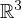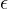Electron. J. Differential Equations, Vol. 2020 (2020), No. 103, pp. 1-34.

### Time periodic solutions for the non-isentropic compressible quantum hydrodynamic equations with viscosity in R^3 Min Li

Abstract:
This article concerns the existence and uniqueness of a time periodic solution for the non-isentropic quantum hydrodynamic equations with viscosity. By applying the Leray-Schauder theory, subtle energy estimates and a limiting method, we obtain the existence of time periodic solutions under some smallness assumptions on the time periodic external force in. The uniqueness can be proved by similar energy estimates. In particular, the quantum effects and the energy equation are taken into account in this paper which play a significant role in the uniform (in the domain R and the positive constant) estimates, especially in the selection of the norm.

Submitted March 29, 2020. Published October 2, 2020.
Math Subject Classifications: 47H11, 35B10, 76Y05, 35Q35, 35G25, 76N10.
Key Words: Time periodic solutions; uniform energy estimates; full quantum hydrodynamic equations with viscosity; Leray-Schauder degree theory.
DOI: 10.58997/ejde.2020.103

Show me the PDF file (437 KB), TEX file for this article.Min Li Faculty of Applied Mathematics Shanxi University of Finance and Economics Taiyuan 030006, China email: minlisxcj@163.com